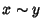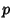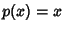## Burnside's Lemma

Letbe a Finite Group and the imagebe a representation which is a Homeomorphism ofinto a Permutation Group, whereis the Group of all permutations of a Set. Define the orbits ofas the equivalence classes under, which is true if there is some permutationinsuch that. Define the fixed points ofas the elementsoffor which. Then the Average number of Fixed Points of permutations inis equal to the number of orbits of.

The Lemma was apparently known by Cauchy(1845) in obscure form and Frobenius (1887) prior to Burnside's (1900) rediscovery. It was subsequently extended and refined by Pólya (1937) for applications in Combinatorial counting problems. In this form, it is known as Pólya Enumeration Theorem.

References

Pólya, G. ``Kombinatorische Anzahlbestimmungen für Gruppen, Graphen, und chemische Verbindungen.'' Acta Math. 68, 145-254, 1937.